Next: About this document ... Up: Converting Al and Be Previous: Modifications for Al

# Traps for the unwary

There are several situations in which the procedure described above will give the wrong results, because they violate the assumptions we've made in simplifying Equation 1:

1. Unexpected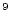Be in sample. We assume that the amount ofBe in the sample is much less than the amount added as carrier, so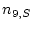can be ignored. Sometimes this isn't true, and the quartz sample contains some Be. This is rare, and almost invariably occurs when the quartz is from a beryl-bearing granite or pegmatite, so there are small amounts of beryl left in the supposedly pure quartz sample. Beryl is not as soluble in HF as quartz, but some of it will dissolve and contribute Be in addition to the Be added as carrier. The only way to detect this is to measure Be as well as Al concentration in the HF solution after sample dissolution, and compare the amount of Be that is actually in the solution to the amount added as carrier.

2. Use of different carriers, or different amounts of carrier. We assume that the number of atoms of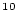Be contributed by the carrier is always the same, so we can simplify by making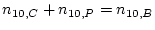. This is the same as assuming that the same carrier solution, and the same amount of carrier solution, is used for all samples and process blanks. If this isn't true,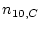andmust be kept separate and estimated individually.

3. Al carrier addition after [Al] measurement. Sometimes there's not enough Al in the quartz sample to extract the minimum amount needed for the AMS measurement, so it's necessary to add some amount of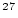Al carrier. If this is added at the time of dissolution, before the aliquot is taken out for [Al] measurement, there is no effect on the procedure described above. The amount of Al you measure in the aliquot is the sum of the Al that was actually in the sample and the Al added as carrier, but you can treat these two sources the same and simply use the total amount of Al as your value for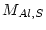. If the Al carrier is added after the [Al] measurement has been made, however, it makes the data reduction much more complicated, and the entire procedure described above must be revised.Next: About this document ... Up: Converting Al and Be Previous: Modifications for Al
2006-05-08